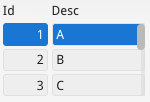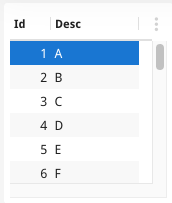# MatrixWhat is a Matrix ?

The term Matrix is not one you will find with an explicit entry in the BDL documentation.  It is the internal name given when elements are repeated in a container that is not intended for repetition i.e not in a TABLE, TREE, or SCROLLGRID.  You may see it inside a .42f, inspecting the AUI Tree,  inside GUI logs etc.

In Informix-4gl it was possible to code …

```SCREEN
{
Id   Desc
[f01][f02     ]
[f01][f02     ]
[f01][f02     ]
}
END

ATTRIBUTES
f01 = formonly.field1;
f02 = formonly.field2;
INSTRUCTIONS SCREEN RECORD scr(field1, field2);```

… note how the item tags f01 and f02 are repeated on multiple lines.  If you compile this form and open the compiled .42f in a text editor you will see …

```<Screen width="15" height="4">
<Label text="Id" posY="0" posX="1" gridWidth="2"/>
<Label text="Desc" posY="0" posX="6" gridWidth="4"/>
<Matrix pageSize="3" name="formonly.field1" colName="field1" fieldId="0" sqlTabName="formonly" tabIndex="1">
<Edit width="3" posY="1" posX="1" gridWidth="3"/>
</Matrix>
<Matrix pageSize="3" name="formonly.field2" colName="field2" fieldId="1" sqlTabName="formonly" tabIndex="2">
<Edit width="8" posY="1" posX="6" gridWidth="8"/>
</Matrix>
</Screen>```

… note how there is a Matrix node wrapped around each field that repeats.

Moving forward with LAYOUT, the same thing occurs if you repeat elements inside a GRID

```LAYOUT (TEXT="Matrix From Layout+Grid")
GRID
{
Id   Desc
[f01][f02     ]
[f01][f02     ]
[f01][f02     ]
}
END
END
ATTRIBUTES
f01 = formonly.field1;
f02 = formonly.field2;
INSTRUCTIONS SCREEN RECORD scr(field1, field2);```

… again you see the Matrix node wrapped around each repeating element …

```<Grid width="15" height="4">
<Label text="Id" posY="0" posX="1" gridWidth="2"/>
<Label text="Desc" posY="0" posX="6" gridWidth="4"/>
<Matrix pageSize="3" name="formonly.field1" colName="field1" fieldId="0" sqlTabName="formonly" tabIndex="1">
<Edit width="3" posY="1" posX="1" gridWidth="3"/>
</Matrix>
<Matrix pageSize="3" name="formonly.field2" colName="field2" fieldId="1" sqlTabName="formonly" tabIndex="2">
<Edit width="8" posY="1" posX="6" gridWidth="8"/>
</Matrix>
</Grid>```

The documentation page on Item tags , discusses the concept of repeated item tags without mentioning the word Matrix.  Look for the phrase “If the same item tag (with the same identifier) appears more than once in the layout,”

Typically we would expect you to use TABLE, SCROLLGRID, or TREE when dealing with repeating elements.  If you ever get puzzled looking at an error message that refers to Matrix, chances are if you look at your form you have a repeating item tag when you shouldn’t, or have meant to code TABLE, SCROLLGRID, or TREE but have coded the container as a GRID.

A Matrix will look like the screenshot on the left, note the small scrollbar.  The screenshot on the right is the same coded as a TABLE. note the better scrollbars, and other GUI decoration such as column headers, alternate row shading.You will find the term Matrix in the Genero Studio documentation.  This is because in the GUI Form Designer you need a way of designing the equivalent of the .per above with repeated elements  This is achieved by adding widgets into a Grid and right-clicking Convert To Matrix to add the repetition.  It is rare that you would need to do this but in the guise of everything you can do in a .per you can do in a .4fd then this is needed.

You can continue to use Matrix and it is something that is needed behind the scenes so that your SCREEN code works in a GUI environment.  You aren’t forced to use TABLE, TREE and SCROLLGRID, but you will get a better user experience if you do.  If you do encounter Matrix, chances are it indicates that you have a repeating element when you don’t mean to, or you are using a GRID container when you perhaps mean to use TABLE, TREE, or SCROLLGRID.